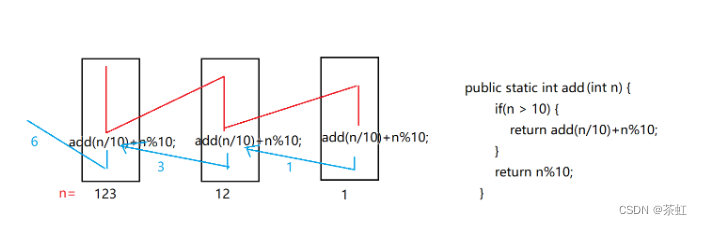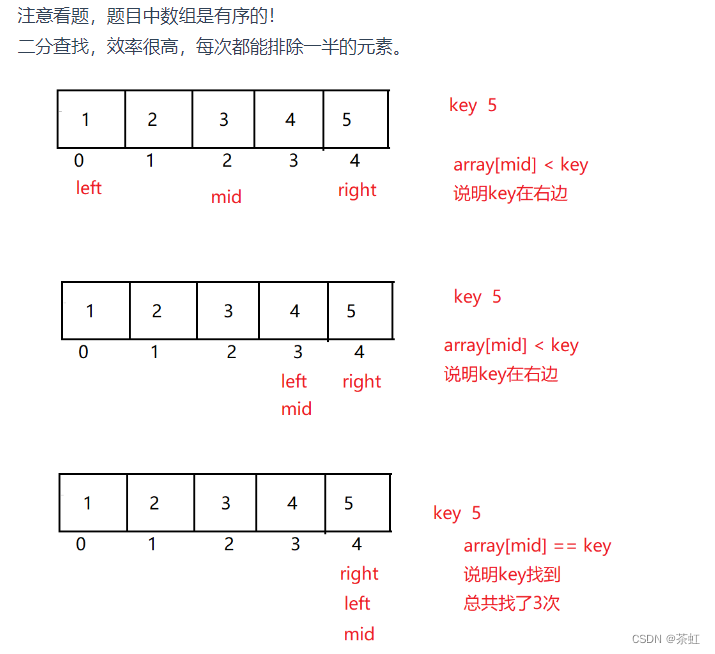# Java---刷题01

33.求和的重载

1. 方法名相同
2. 参数列表不同（数据类型，个数，顺序）
3. 返回值无关
``````public static int sum(int a,int b) {
return a+b;
}

public static double sum(double a,double b,double c) {
return a+b+c;
}``````

34.求1！+2！+3！+4！+........+n!的和

`````` /**

* 1!+2!+3!+.....+n!

* @param n

* @return

*/

public static int facSum(int n) {

int sum = 0;

for (int i = 1; i <= n; i++) {
//fac为 求指定数字的阶乘的函数
sum += fac(i);

}

return sum;

}

/**

* 求i!的阶乘

* @param i

* @return

*/

public static int fac( int i) {

int tmp = 1;

for (int j = 1; j <= i; j++) {

tmp *= j;

}

return tmp;

}

public static void main(String[] args) {

int ret = facSum(5);

System.out.println(ret);

}``````

35.找出出现一次的数字

``````class Solution {
vector<int> onlyones(vector<int>nums) {

int count = 0;
int i = 0;
int cur=nums[i];
vector<int>res;
while (i<nums.size()) {
if (nums[i] == cur) {
count++;
if (count == 2) {
cur = nums[++i];
count = 1;
}
i++;
}
else {
res.push_back(cur);
cur = nums[i++];
count = 1;
}
}
return res;
}
};``````

36.求最大值方法的重载

1. 方法名相同
2. 参数列表不同（数据类型，个数，顺序）
3. 返回值无关

Math的使用 不需要导入相关的包

``````public static int max(int a,int b) {
return Math.max(a,b);
}

public static double max(double a,double b,double c) {

double m = Math.max(a,b);
return Math.max(m,c);
}
``````

37.求 N 的阶乘

``````public static int fac( int n) {

int tmp = 1;

for (int j = 1; j <= n; j++) {

tmp *= j;

}

return tmp;

}``````

38.求斐波那契数列的第n项。(迭代实现)

``````/**

* 求菲薄那切数列的第n项

* @param n

* @return

*/

public static int fib(int n) {
if(n == 1 || n == 2 ) {
return 1;
}
int f1 = 1;

int f2 = 1;

int f3 = 1;

for (int i = 3; i <= n; i++) {

f3 = f1+f2;

f1 = f2;

f2 = f3;

}

return f3;

}
``````

39.写一个递归方法，输入一个非负整数，返回组成它的数字之和``````public static int add(int n) {
if(n > 10) {
}
return n%10;
}``````

40.递归求斐波那契数列的第 N 项

`````` public static int fib(int n) {

if(n == 1 || n == 2) {

return 1;

}

return fib(n-1)+fib(n-2);

}``````

41.按顺序打印一个数字的每一位(例如 1234 打印出 1 2 3 4) （递归）

123 相当于先打印12的每一位，再打印3

12 相当于先打印1的每一位，再打印2

1 只有一位数，直接打印。

``````public static void print(int n) {
if(n <= 9) {
System.out.println(n%10);
return;
}
print(n/10);
System.out.println(n%10);
}
``````

42.递归求 1 + 2 + 3 + ... + 10

``````public static int add(int n) {

if(n == 1) {
return 1;
}
}``````

43.递归求 N 的阶乘

``````/**
* 阶乘的代码！

* 递归的优点：代码量少
* @param n

* @return

*/

public static int fac(int n) {
if(n == 1) {
return 1;
}
return n * fac(n-1);
}
``````

44.改变原有数组元素的值

``````//方式一：扩大的还是原来的数组，这样做的不好的地方是，改变了原来数组的值
public static void func2(int[] array) {

for (int i = 0; i < array.length; i++) {

array[i] = array[i]*2;

}

}

//方式二：扩大的新的数组，没有修改原来的值
public static int[] func3(int[] array) {

int[] tmp = new int[array.length];//

for (int i = 0; i < array.length; i++) {

tmp[i] = array[i]*2;

}

return tmp;

}``````

45.创建一个 int 类型的数组, 元素个数为 100, 并把每个元素依次设置为 1 - 100

``````public static void main(String[] args) {
int[] array = new int;
for (int i = 0; i < array.length; i++) {
//题目要求赋值1-100，这里利用下标的值，然后+1即可。
array[i] = i+1;
}
}``````

46.两数之和

``````public int[] twoSum(int[] nums, int target) {
int[] result = new int;
// 双指针i和j，i从前向后遍历，j从后向i遍历，若arr[i]+arr[j]=target,即为题解
for (int i = 0; i < nums.length - 1; i++) {
for (int j = nums.length - 1; j > i; j--) {
if (nums[i] + nums[j] == target) {
result = i;
result = j;
}
}
}
return result;
}``````

47.给定一个整型数组, 实现冒泡排序(升序排序)

``````public static void bubbleSort(int[] array) {
boolean flg = false;
//1、确定一个趟数
for (int i = 0; i < array.length-1; i++) {
for (int j = 0; j < array.length-1-i; j++) {
if(array[j] > array[j+1]) {
int tmp = array[j];
array[j] = array[j+1];
array[j+1] = tmp;
flg = true;
}
}
if(flg == false) {
//没有交换
break;
}
}
}``````

48.给定一个整型数组, 判定数组是否有序（递增）

49.定一个有序整型数组, 实现二分查找``````public static int binarySearch(int[] array,int key) {
int left = 0;
int right = array.length-1;
while (left <= right) {
int mid = (left+right)/2;
if(array[mid] == key) {
return mid;
}else if(array[mid] < key) {
left = mid+1;
}else {
right = mid-1;
}
}
return -1;//代表没有找到这个数字
}``````

50.实现一个方法 copyOf, 对一个整型数组进行拷贝, 得到一个新的数组.

### 网站公告